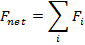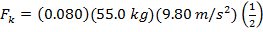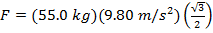# Kinetic Friction Formula

Kinetic Friction Formula

Kinetic friction is a force that acts between moving surfaces. An object that is being moved over a surface will experience a force in the opposite direction as its movement. The magnitude of the force depends on the coefficient of kinetic friction between the two kinds of material. Every combination is different. The coefficient of kinetic friction is assigned the Greek letter "mu" (μ), with a subscript "k". The force of kinetic friction is μk times the normal force on an object, and is expressed in units of Newtons (N).

force of kinetic friction = (coefficient of kinetic friction)(normal force)

Fk = μk η

Fk = force of kinetic friction

μk = coefficient of kinetic friction

η = normal force (Greek letter "eta")

Kinetic Friction Formula Questions:

1) A worker in a stock room pushes a large cardboard box across the floor. The coefficient of kinetic friction is μk = 0.520. The box has a mass of 75.0 kg, and the worker is exerting a force of 400.0 Nforward. What is the magnitude of the force of friction, and what is the net force moving the box?

Answer: On a flat surface, the normal force on an object is η = mg. Using this, the formula can be used to find the force of friction:

Fk = μk η

Fk = μk mg

Fk = (0.520)(75.0 kg)(9.80 m/s2)

Fk = 382.2 kg∙m/s2

Fk = 382.2 N

The force of kinetic friction acting in the opposite direction as the motion of the box is 382.2 N. The net force acting on the box is the sum of forces. The two forces to consider are the force of kinetic friction acting in the opposite direction as the box's motion, and the force exerted by the worker, which is 400 N forward. If we define "forward" as the positive direction, the net force is:Fnet=Fworker-Fk

Fnet=400.0 N-382.2N

Fnet = 17.8 N

The net force acting on the box is 17.8 N forward.

2) A woman is skiing straight down a snow-covered hill. The coefficient of kinetic friction between the skis and the snow is μk = 0.0800. The hill is at a 60.0° angle from the horizontal. The skier's mass is 55.00 kg. What is the magnitude of the force of kinetic friction, and what is the net force along the skier's direction of motion?

Answer: On a flat surface, the normal force on an object is η = mg. On a surface that is at an angle relative to the horizontal axis, The total force due to gravity, F = mg, must be broken in to components. The normal force is the component that is perpendicular to the angled surface, and the remaining force is parallel to the angled surface. The normal force is η = mg cosθ, and the remaining force component is F = mg sinθ. Using this, the formula can be used to find the magnitude of the force of kinetic friction:

Fk = μk η

Fk = μk mg cosθ

Fk = (0.0800)(55.00 kg)(9.80 m/s2)cos(60°)Fk = 21.6 kg∙m/s2

Fk = 21.6 N

The force of kinetic friction opposes the motion of the skier. The force that moves the skier down the hill is the remaining component of the force of gravity, Fθ = mg sinθ. This force is:

F = mg sinθ

F =(55.0 kg)(9.80 m/s2)sin(60°)F = 466.8kg∙m/s2

F = 466.8N

The net force acting on the skier is the sum of forces. The two forces to consider are the force directed down the hill and the force of kinetic friction directed up the hill. If we define the positive direction as down the hill, which is the skier's direction of motion, the net force is:Fnet = F-Fk

Fnet = 466.8 N-21.6 N

Fnet = 445.2 N

The net force acting on the skier in her direction of motion is on the box is 445.2 N.

 Related Links: Potential & Kinetic Energy Quiz Chemical Kinetics and Rates Quiz Kinetic Energy Examples Kinetic Energy Formula Inertia Examples Rolling Friction Examples Static Friction Formula Stopping Distance Formula